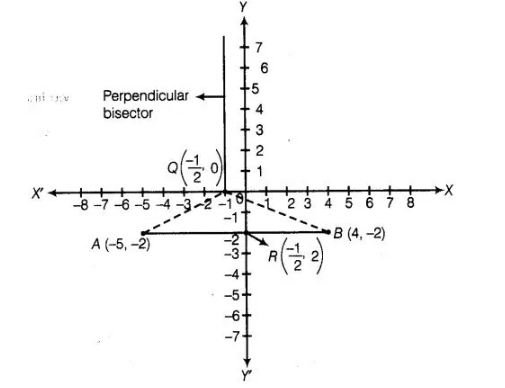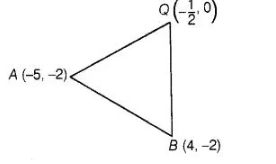# Find the coordinates of the point Q on the x-axis

Question:

Find the coordinates of the point Q on the x-axis which lies on the perpendicular bisector of the line segment joining the points A (- 5, – 2) and B (4, – 2).

Name the type of triangle formed by the point Q, A and B.

Solution:

Firstly, we plot the points of the line segment on the paper and join them.We know that, the perpendicular bisector of the line segment AB bisect the segment AB, i.e.,perpendicular bisector of the line segment AB passes

through the mid-point of AB.

$\therefore \quad$ Mid-point of $A B=\left(\frac{-5+4}{2}, \frac{-2-2}{2}\right)$

$\Rightarrow \quad R=\left(-\frac{1}{2},-2\right)$

$\left[\because\right.$ mid-point of a line segment passes through the points $\left(x_{1}, y_{1}\right)$ and $\left(x_{2}, y_{2}\right)$ is

Now, we draw a straight line on paper passes through the mid-point $R$. We see that perpendicular bisector cuts the $X$-axis at the point $Q\left(-\frac{1}{2}, 0\right)$.

Hence, the required coordinates of $Q \equiv\left(-\frac{1}{2}, 0\right)$

Alternate Method                                                                                              •

(i) To find the coordinates of the point of 0 on the X-axis. We find the equation of perpendicular bisector of the line segment AS.

Now, slope of line segment AB,

Let   $m_{1}=\frac{y_{2}-y_{1}}{x_{2}-x_{1}}=\frac{-2-(-2)}{4-(-5)}=\frac{-2+2}{4+5}=\frac{0}{9}$

$\Rightarrow \quad m_{1}=0$

Let the slope of perpendicular bisector of line segment is $m_{2}$.

Since, perpendicular bisector is perpendicular to the line segment $A E$.

By perpendicularity condition of two lines,

$m_{1}: m_{2}=-1$

$\Rightarrow$ $m_{2}=\frac{-1}{m_{1}}=\frac{-1}{0}$

$\Rightarrow$ $m_{2}=\infty$

Also, we know that, the perpendicular bisector is always passes through the mid-point of the line segment.

$\therefore$ Mid-point $=\left(\frac{-5+4}{2}, \frac{-2-2}{2}\right)=\left(\frac{-1}{2},-2\right)$ $\left[\because\right.$ mid-point

$\left.=\left(\frac{x_{1}+x_{2}}{2}, \frac{y_{1}+y_{2}}{2}\right)\right]$

To find the equation of perpendicular bisector of line segment, we find the slope and a point through which perpendicular bisector is pass.

Now, equation of perpendicular bisector having slope $\infty$ and passing through the point

$\left(\frac{-1}{2},-2\right)$ is,

$(y+2)=\infty\left(x+\frac{1}{2}\right) \quad\left[\because\left(y-y_{1}\right)=m_{2}\left(x-x_{1}\right)\right]$

$\Rightarrow$ $\frac{y+2}{x+\frac{1}{2}}=\infty=\frac{1}{0} \Rightarrow x+\frac{1}{2}=0$

$\therefore$ $x=\frac{-1}{2}$

So, the coordinates of the point $Q$ is $\left(\frac{-1}{2}, 0\right)$ on the $X$-axis which lies on the perpendicular

bisector of the line segment joining the point $A B$.

To know the type of triangle formed by the points $Q, A$ and $B$. We find the length of all three sides and see whatever condition of triangle is satisfy by these sides.

Now, using distance formula between two points,

$A B=\sqrt{(4+5)^{2}+(-2+2)^{2}}=\sqrt{(9)^{2}+0}=9$

$\left[\because\right.$ distance between two points $\left(x_{1}, y_{1}\right)$ and $\left.\left(x_{2}, y_{2}\right)=\sqrt{\left(x_{2}-x_{1}\right)+\left(y_{2}-y_{1}\right)^{2}}\right]$

$B Q=\sqrt{\left(\frac{-1}{2}-4\right)^{2}+(0+2)^{2}}$

$=\sqrt{\left(\frac{-9}{2}\right)^{2}+(2)^{2}}=\sqrt{\frac{81}{4}+4}=\sqrt{\frac{97}{4}}=\sqrt{\frac{97}{2}}$

and $Q A=\sqrt{\left(-5+\frac{1}{2}\right)+(-2-0)^{2}}$

$=\sqrt{\left(\frac{-9}{2}\right)^{2}+(2)^{2}}$

$=\sqrt{\frac{81}{4}+4}=\sqrt{\frac{97}{4}}=\sqrt{\frac{97}{2}}$

We see that, $B Q=Q A \neq A B$which shows that the triangle formed by the points Q, A and 6 is an isosceles.